# Set Operations

The set operations are performed on two or more sets to obtain a combination of elements as per the operation performed on them. In a set theory, there are three major types of operations performed on sets, such as:

1. Union of sets (∪)
2. Intersection of sets (∩)
3. Difference of sets ( – )

Let us discuss these operations one by one.

## Union of Sets

If two sets A and B are given, then the union of A and B is equal to the set that contains all the elements present in set A and set B. This operation can be represented as;

A ∪ B = {x: x ∈ A or x ∈ B}

Where x is the elements present in both sets A and B.

Example: If set A = {1,2,3,4} and B {6,7}

Then, Union of sets, A ∪ B = {1,2,3,4,6,7}

Venn Diagram of Union of sets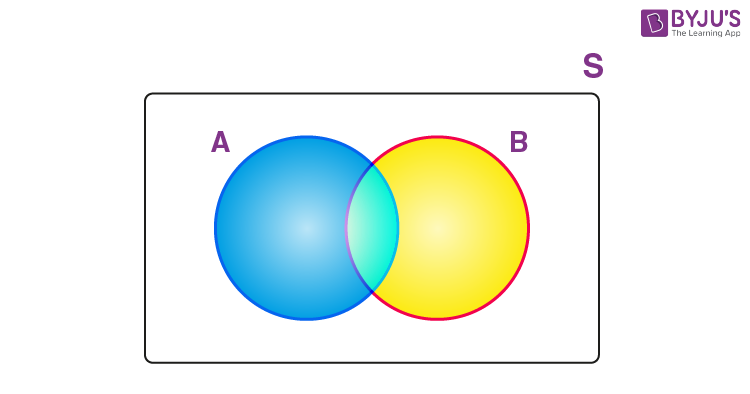## Intersection of Sets

If two sets A and B are given, then the intersection of A and B is the subset of universal set U, which consist of elements common to both A and B. It is denoted by the symbol ‘∩’. This operation is represented by:

A∩B = {x : x ∈ A and x ∈ B}

Where x is the common element of both sets A and B.

The intersection of sets A and B, can also be interpreted as:

A∩B = n(A) + n(B) – n(A∪B)

Where,

n(A) = cardinal number of set A,

n(B) = cardinal number of set B,

n(A∪B) = cardinal number of union of set A and B.

Example: Let A = {1,2,3} and B = {3,4,5}

Then, A∩B = {3}; because 3 is common to both the sets.

Venn Diagram of Intersection of sets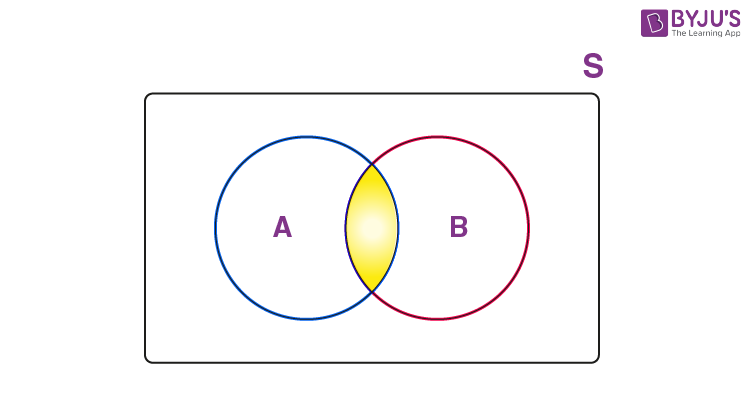Intersection of Two Sets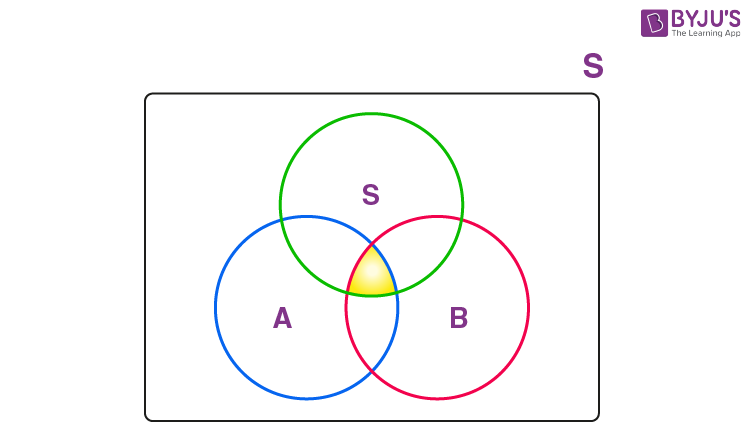Intersection of three sets

## Difference of Sets

If there are two sets A and B, then the difference of two sets A and B is equal to the set which consists of elements present in A but not in B. It is represented by A-B.

Example: If A = {1,2,3,4,5,6,7} and B = {6,7} are two sets.

Then, the difference of set A and set B is given by;

A – B = {1,2,3,4,5}

We can also say, that the difference of set A and set B is equal to the intersection of set A with the complement of set B. Hence,

A−B=A∩B

Venn Diagram of Difference of sets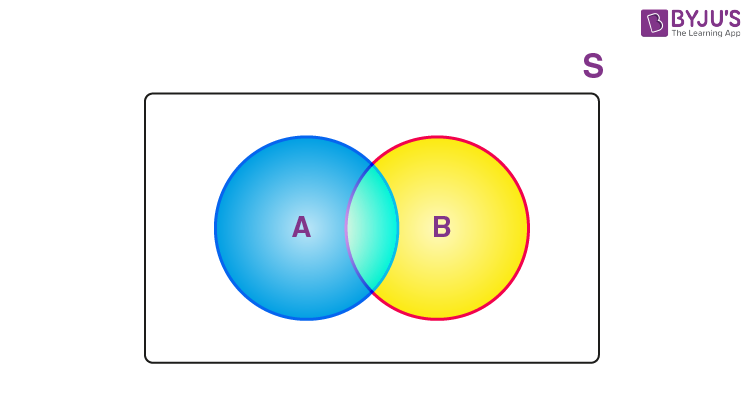### Complement of Set

If U is a universal set and X is any subset of U then the complement of X is the set of all elements of the set U apart from the elements of X.

X′ = {a : a ∈ U and a ∉ A}

Venn Diagram: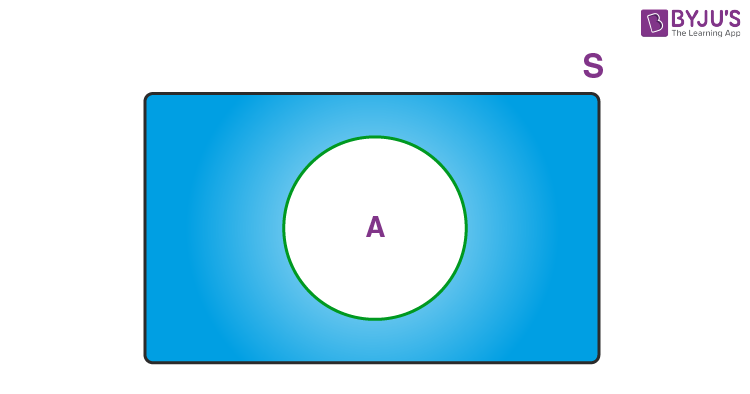Example: U = {1,2,3,4,5,6,7,8}

A = {1,2,5,6}

Then, complement of A will be;

A’ = {3,4,7,8}

## Video Lesson on What are Sets## Properties of Set Operations

Commutative Property

A ∪ B = B ∪ A

A ∩ B = B ∩ A

Associative Property

A ∪ (B ∪ C) = (A ∪ B) ∪ C

A ∩ (B ∩ C) = (A ∩ B) ∩ C

Distributive Property

A ∩ (B ∪ C) = (A ∩ B) ∪ (A ∩ C)

A ∪ (B ∩ C) = (A ∪ B) ∩ (A ∪ C)

Test your knowledge on Set Operations